# Equations Math problems

#### Number of problems found: 1601

• Equation with absSolve this equation with absolute value member: ?
• Simple equation 5Solve equation with fractions: X × 3/8 = 1/2
• Variation equation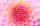Solve combinatorics equation: V(2, x+8)=72
• Simple equationSolve the following simple equation: 2. (4x + 3) = 2-5. (1-x)
• Simple equation 2Find X in this simple equation: X/9 = 96/108
• Roots count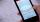Substitute the numbers/0,1,2,3/into the equation as x: (x - 1) (x - 3) (x + 1) = 0 Which of them is its solution? Is there another number that solves this equation?
• Diofant equationIn the set of integers (Z) solve the equation: ? Write result with integer parameter ? (parameter t = ...-2,-1,0,1,2,3... if equation has infinitely many solutions)Solve quadratic equation: 2x2+28x-550=0
• EquationSolve equation and check the result: 1.4x - 3/2 + x - 9,8 = x + 0,4/3 - 7 + 1,6/6
• RootsDetermine the quadratic equation absolute coefficient q, that the equation has a real double root and the root x calculate: ?Quadratic equation ? has roots x1 = -26 and x2 = -86. Calculate the coefficients b and c.
• Equation algebraogram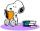Solve the equation: oco + ivo = cita How much has the task of solutions?
• VCP equationSolve the following equation with variations, combinations and permutations: 4 V(2,x)-3 C(2,x+ 1) - x P(2) = 0
• Hyperbola equationFind the hyperbola equation with the center of S [0; 0], passing through the points: A [5; 3] B [8; -10]
• Equation 11Solve equation: ?Find the roots of the quadratic equation: 3x2-4x + (-4) = 0.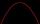Solve pure quadratic equation ?.
• Equation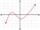Eequation f(x) = 0 has roots x1 = 64, x2 = 100, x3 = 25, x4 = 49. How many roots have equation f(x2) = 0 ?Determine the numbers b, c that the numbers x1 = -1 and x2 = 3 were roots of quadratic equation: ?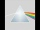Please determine the solvability conditions of the equation, solve the equation and perform the test: x divided by x squared minus 2x plus1 the whole minus x + 3 divided by x squared minus 1 this is equal to 0: x/(x2-2x+1) - (x+3)/( x2-1) = 0